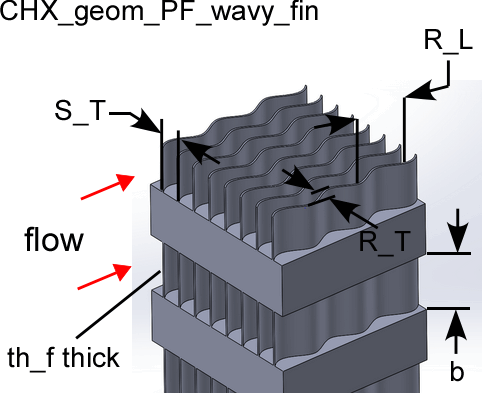Contents - Index

PF wavy-fin geometryThe procedure:

CHX_geom_pf_wavy_fin(TypeHX\$: S_T, th_f, b, R_T, R_L, D_h, theta, beta, A_fin\A)

Provides the geometric data associated with a plate finned - wavy-fin type compact heat exchanger surface.  These data are from Kays and London (1994).

Inputs:

The only input is the string identifying the geometry TypeHX\$

11.44-3/8W:  'PF_wavy-fin_1144_38w'

11.5-3/8W: 'PF_wavy-fin_115_38w'

17.8-3/8W: 'PF_wavy-fin_178_38w'

Outputs:

S_T = transverse fin spacing (m or ft)

th_f = thickness of fin material (m or ft)

b = plate gap (m or ft)

R_T = tranverse ripple amplitude (m or ft)

R_L = longitudinal ripple amplitude (m or ft)

D_h = hydraulic diameter defined as 4 x minimum flow area x length/heat transfer area (m or ft)

theta = minimum flow area/frontal area for finned surface side only

beta = heat transfer area/volume (m^2/m^3 or ft^2/ft^3) for finned surface side only

A_fin\A = heat transfer area on fins/heat transfer area for finned surface side only

Example

\$UnitSystem SI Mass J K Pa

TypeHX\$= 'PF_wavy-fin_115_38W'

Call chx_geom_pf_wavy_fin(TypeHX\$: S_T, th_f, b, R_T, R_L, D_h, theta, beta, A_fin\A)

{Solution is:

S_T = 0.00221 [m]

th_f = 0.000254 [m]

b = 0.00925 [m]

R_T = 0.001981 [m]

R_L = 0.009525 [m]

D_h = 0.003023 [m]

theta = 0.860 [-]

beta = 1138 [m^2/m^3]

A_fin\A = 0.822}

Related procedures include:

Nondimensional Functions

Coefficient of Heat Transfer

Pressure Drop

Compact Heat Exchangers